American Association of Physics Teachers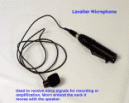Jorge Pullin's TALK  -- p. 1 A Brief Reminder on General Relativity from the AAPT Topical Workshop: Teaching General Relativity to Undergraduates Syracuse University, July 20-21, 2006Jorge Pullin The graphics from Pullin's talk are here in seven web pages. Click on the indicated subject to go to the relevant page. You can also download a PDF version if you wish. pg. 1 Pullin began the workshop with a succinct account of GR.  He pointed participants to more information in Wikipedia, noting especially Sean Carroll's no-nonsense introduction, and then he outlined his talk.    pg. 2  He gave a one-slide summary of the special relativity needed to understand general relativity, and then showed the essence of GR: gravity is curvature of spacetime and the spacetime interval is the measure of "distance". pg. 3 To describe curved space you need tensors. Trajectories are curves parameterized by the proper time, and absent forces, these are straight lines in spacetime. For vector algebra you need a metric; for vector calculus you need the covariant derivative. pg. 4 Einstein's equations tell how matter curves spacetime.  The curvature affects physics. You need to know how curvature shows up in the mathematics: Ricci tensor and curvature scalar.  Minimal coupling relates physics to curved space; he shows the weak field metric outside a sphere. pg. 5  There are singularities in this metric. One is an artifact of choice of coordinates; the other implies there are black holes. In the weak field limit there is an effective potential that accounts for the precession of the perihelion of Mercury.  This effective potential is an "exterior" solution, not valid in close. pg. 6 The weak-field metric implies there should be gravity waves. The possibility of such waves can also be seen by using Kruskal coordinates and obtaining a wave equation. He then uses a Green's function to obtain a solution of this wave equation. pg. 7 Summary: Gravity is the deformation of geometry; Einstein's equations describe how geometry is deformed; solutions of these equations describe well the effects of weak fields and predict new phenomena for strong fields; the equations have wave-like solutions, but the lowest contribution is quadrupolar.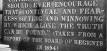wiki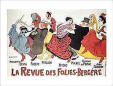review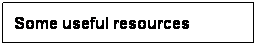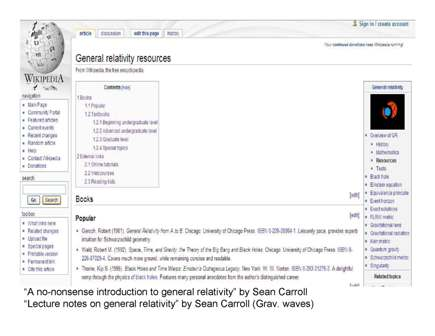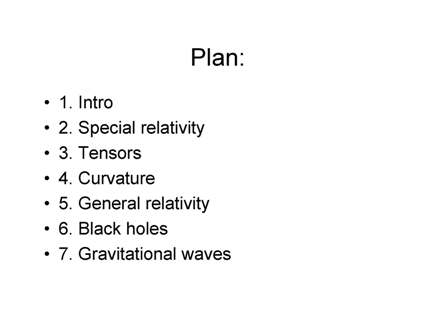links:      Home         Suggest website improvements at AAPT's Wiki                  AAPT                     AJP                      TPT                  PSRC                ComPADRE

mumbo jumbo luxor. site about luxor amun.